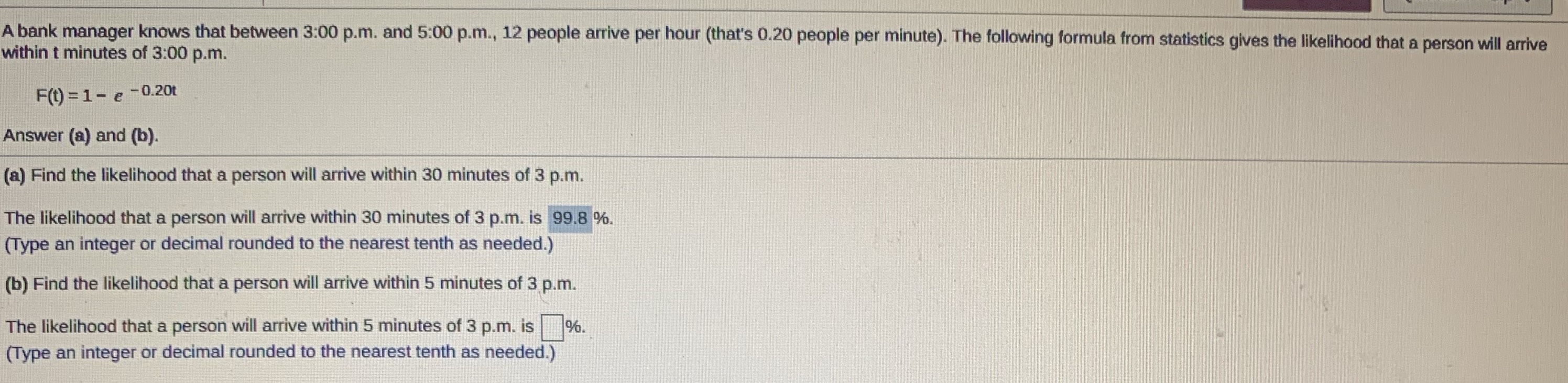### ¿Todavía tienes preguntas de matemáticas?

Pregunte a nuestros tutores expertos
Algebra
PreguntaA bank manager knows that between 3:00 p.m. and 5:00 p.m., $$12$$ people arrive per hour (that's $$0.20$$ people per minute). The following formula from statistics gives the likelihood that a person will arrive within t minutes of $$3 : 00$$ p.m. $$F ( t ) = 1 - e ^ { - 0.20 t }$$. Answer (a) and $$( b )$$ ,(b) Find the likelihood that a person will arrive within $$5$$ minutes of $$3$$ p.m. The likelihood that a person will arrive within $$5$$ minutes of $$3$$ p.m. is $$\square \%$$ . (Type an integer or decimal rounded to the nearest tenth as needed.)